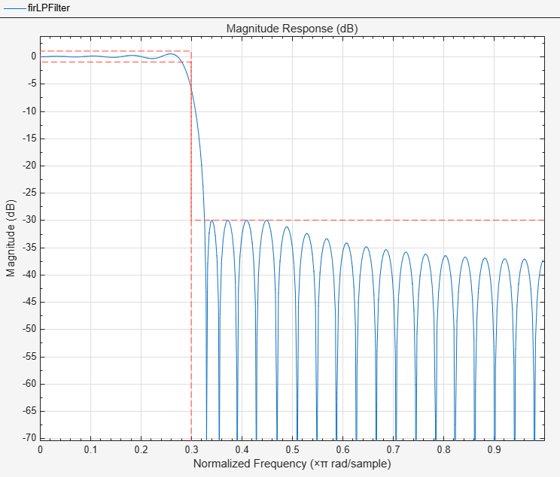# fircls

FIR Constrained Least Squares filter

## Syntax

```clsFilter = design(d,'fircls','SystemObject',true) clsFilter = design(d,'fircls','FilterStructure',value,'SystemObject',true) clsFilter = design(d,'fircls','PassbandOffset',value,'SystemObject',true) clsFilter = design(d,'fircls','zerophase',value,'SystemObject',true) ```

## Description

`clsFilter = design(d,'fircls','SystemObject',true)` designs a FIR Constrained Least Squares (CLS) filter, `clsFilter`, from a filter specifications object, `d`.

`clsFilter = design(d,'fircls','FilterStructure',value,'SystemObject',true)` where `value` is one of the following filter structures:

• `'dffir'`, a discrete-time, direct-form FIR filter (the default value)

• `'dffirt'`, a discrete-time, direct-form FIR transposed filter

• `'dfsymfir'`, a discrete-time, direct-form symmetric FIR filter

`clsFilter = design(d,'fircls','PassbandOffset',value,'SystemObject',true)` where `value` sets the passband band gain in dB. The `PassbandOffset` and `Ap` values affect the upper and lower approximation bound in the passband as follows:

• Lower bound = (`PassbandOffset`-`Ap`/2)

• Upper bound = (`PassbandOffset`+`A`/2)

For bandstop filters, the `PassbandOffset` is a vector of length two that specifies the first and second passband gains. The `PassbandOffset` value defaults to 0 for lowpass, highpass and bandpass filters. The `PassbandOffset` value defaults to [0 0] for bandstop filters.

`clsFilter = design(d,'fircls','zerophase',value,'SystemObject',true)` where `value` is either `'true'` ('1') or `'false'` ('0') . If `zerophase` is true, the lower approximation bound in the stopband is forced to zero (i.e., the filter has a zero phase response). Zerophase is false (0) by default.

To determine the available design options, use `designopts` with the specification object and the design method as input arguments as shown.

`designopts(d,'fircls')`

For complete help about using `fircls`, refer to the command line help system. For example, to get specific information about using `fircls` with `d`, the specification object, enter the following at the MATLAB prompt.

`help(d,'fircls')`

## Examples

collapse all

The following example designs a constrained least-squares FIR lowpass filter.

```h = fdesign.lowpass('n,fc,ap,ast', 50, 0.3, 2, 30); firLPFilter = design(h, 'fircls','SystemObject',true); fvtool(firLPFilter)```The following example constructs a constrained least-squares FIR bandpass filter.

```d = fdesign.bandpass('N,Fc1,Fc2,Ast1,Ap,Ast2',... 30,0.4,0.6,60,1,60); firBPFilter = design(d,'fircls','SystemObject',true); fvtool(firBPFilter)```